## CTFS Tutorials

Source file: http://ctfs.si.edu/ctfsdev/CTFSRPackageNew/files/tutorials/growthfitAnalysis Modeling AGB growth by optimizing dbh bins

Growth vs. DBH

## MODELING AGB GROWTH BY OPTIMIZING DBH BINS

Date: April 7, 2011.

The following calculations in R run a model of AGB growth as a function of diameter, or as a function of agb, using a series of discrete size categories and a linear regression within each. The model presented here uses log(dbh) or log(agb) as the predictor (independent variable). The locations of the categories, or bins, of log(dbh) are not defined in advance, but are fitted as part of the model.

The R commands listed show all steps from loading data to running the model and graphing the output. All commands begin with >, and if continued on a second line, +. Comments between the commands describe the input and output of each.

The calculations start with the full data dataframes. These are described at
http://ctfs.arnarb.harvard.edu/yourplot/RAnalyticalTables (of course you have to substitute a plot name for yourplot).

### 1. Source necessary functions

The following packages are necessary:

• growth.r
• growthfit.bin.r
• utilities.r
• statistics.r
• distributions.r

Running startCTFS will load these. The argument folder must match the location of the package on your computer.

> source('/home/condit/CTFSRPackage/startCTFS/startCTFS.r')
> startCTFS(folder='/home/condit/CTFSRPackage/')

Census data can be loaded using the function CTFSplot in utilities.r (or just attach the files). In the example here, there must be files bci.full5 and bci.full6 inside a folder full inside the listed path (so /home/fullplotdata/full/bci.full5 and same for bci.full6).

> CTFSplot('bci',5:6,type='full',path='/home/fullplotdata/')

### 3. Calculate growth rates

3.1. Create a table of biomass growth rates using the function extract.growth.

The following creates a table of AGB increment for every individual with dbh100 mm. It excludes trees growing faster than 75 mm yr1 (in dbh growth), or shrinking by 4 x the measurement error (which is 1 mm in small trees, 4-6 mm in big trees). Diameter is log-transformed (using logit=’x’). These are appropriate options for the calculations that follow. If you are worried about trimming the outliers, check the functions graph.outliers and graph.outliers.spp in order to see what is excluded. You need to change dbhunit to cm and maxgrow to 7.5 if your dbh is in centimeters, and set mindbh=10.

Of course you should submit census tables from your own plot instead of bci.full5 and bci.full6.

Note that I occasionally use if(!exists). You can ignore these when executing functions. I use it to prevent certain long-running commands from repeating every time I test the calculations. In this case, once agb.growth is created, it won’t be created again.

> cns1=bci.full5
> cns2=bci.full6
> if(!exists('agb.growth'))
+   agb.growth=extract.growthdata(census1=cns1,census2=cns2,growcol='incgr',
+                                 growthfunc=growth.biomass.indiv,logit='x',rounddown = FALSE,
+                                 mindbh = 100,dbhunit = 'mm',err.limit = 4,maxgrow = 75)

sp treeID      dbh        agb        growth
19 gustsu     19 5.697093 -0.3739816  0.0071891093
21 virosu     21 5.852202 -0.3032694  0.0098357161
23 quaras     23 5.749393 -0.4840976 -0.0009974601
24 protte     24 6.082219  0.5892221  0.0378020363
25 brosal     25 7.162397  3.1977491  0.0000000000
30 quaras     30 6.146329  0.5224756  0.0465738782

3.2. Graphing growth rate.

This extracts growth for just one species. You might also make a graph of all growth rates at once in order to explore the data.

> onespdata=subset(agb.growth,sp=='tet2pa')
> plot(onespdata\$dbh,onespdata\$growth,pch=16,xlab='log(dbh)',ylab='AGB growth increment')

Figure 3.1: AGB growth rate of individual trees of Tetragastris panamensis as a function of log(dbh). BCI, 2000-2005. Note the few negative growth rates which remained. Only extreme negatives are trimmed.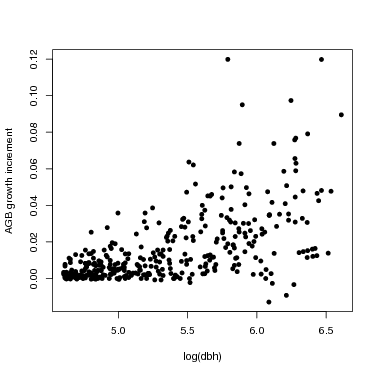### 4. The model

4.1. Fitting.

Here is how to fit the model for just a single species, then graph the result. The table onespdata produced above has growth data from a single species that is appropriate for the function. For testing, you can start by using method=optim, since it produces the fit faster, but optim does not produce confidence limits, so use method=Gibbs for final results.

Here, dbh is used as the predictor in the model, but this can be changed by setting sizecol=’agb’ instead of dbh.

The option nobin sets how many dbh categories will be used. Here it is set to 2, so just one division point will be estimated. (The location of the division point will be optimized, but the number of division points must be set at the start.)

The option sdmodel defines the model used for related SD to log(size). The default is linear.model.ctr, simply a straight line with intercept defined at the mid-point of the x axis. The number of parameters for the SD model, defined by startsd, must match the number of parameters expected by sdmodel: 2 parameters for a linear model. The second run here (fit2) illustrates a model with 3 parameters, where the SD is constant below a certain value of x, then increases linearly. This allows the SD to increase rapidly at large size while remaining positive at small size.

> if(!exists('fit'))
+  fit=growth.flexbin(growthtable=onespdata,sizecol='dbh',nobin=2,start=NULL,startsd=c(.02,0),
+                     sdmodel=linear.model.ctr,method='Gibbs',rep=1500,burn=500,show=100)
> data.frame(names(fit))

names.fit.
1        best
2    bestmean
3          CI
4      bestSD
5  bestSDmean
6      confSD
7       model
8   fullparam
9       sdpar
10      llike
11       burn
12       keep
13 llike.best
14     growth
15       bins
16   optimpar
17    optimSD
18 optimllike
19  max.param

> if(!exists('fit2'))
+  fit2=growth.flexbin(growthtable=onespdata,sizecol='dbh',nobin=2,start=NULL,startsd=c(.02,0,.001),
+                     sdmodel=constant.linear,method='Gibbs',rep=1500,burn=500,show=100)

4.2. Model output.

The output of the model was saved in the variables fit and fit2, which are lists that include a lot of information. The element fit\$model includes the original data (fit\$model\$x and fit\$model\$y), plus the fitted value for each (fit\$model\$pred), and also the fitted SD at every point (fit\$model\$predSD). The best fit model parameters are fit\$best, and confidence limits in fit\$CI. The best fit standard deviation parameters are in fit\$bestSD. (The model assumes a linear increase in the standard deviation around the line, and the two SD parameters describe the linear increase.)

> fit\$best

 5.59861462 0.01587726 0.03044343 0.01274679

> fit\$CI

[,1]       [,2]       [,3]       [,4]
2.5%  5.182171 0.01109343 0.01427356 0.01025213
97.5% 6.350274 0.01907803 0.13527083 0.01433389

x            y        pred      predSD
41192  4.605170 0.0024277875 0.002143495 0.002587984
219538 4.605170 0.0033515898 0.002143495 0.002587984
21229  4.615121 0.0067920171 0.002301479 0.002733811
33563  4.615121 0.0077488471 0.002301479 0.002733811
133039 4.615121 0.0002578913 0.002301479 0.002733811
163857 4.615121 0.0007855686 0.002301479 0.002733811

> par(mfcol=c(2,1),mai=c(1,1,0.25,0.25),cex.lab=1.5,cex.axis=1.5)
> plot(fit\$model\$x,fit\$model\$y,pch=16,xlab='log(dbh)',ylab='AGB growth')
> lines(fit\$model\$x,fit\$model\$pred,col='red',lwd=2)
> lines(fit\$model\$x,fit\$model\$pred+fit\$model\$predSD,col='green',lwd=2)
> lines(fit\$model\$x,fit\$model\$pred-fit\$model\$predSD,col='green',lwd=2)
> plot(fit2\$model\$x,fit2\$model\$y,pch=16,xlab='log(dbh)',ylab='AGB growth')
> lines(fit2\$model\$x,fit2\$model\$pred,col='red',lwd=2)
> lines(fit2\$model\$x,fit2\$model\$pred+fit2\$model\$predSD,col='green',lwd=2)
> lines(fit2\$model\$x,fit2\$model\$pred-fit2\$model\$predSD,col='green',lwd=2)

Figure 4.1: Fit of the two-bin model to growth date for Tetragastris panamensis at BCI. The red line shows fit\$model\$pred, the fitted growth rate. The green lines are one fitted SD above and below the line.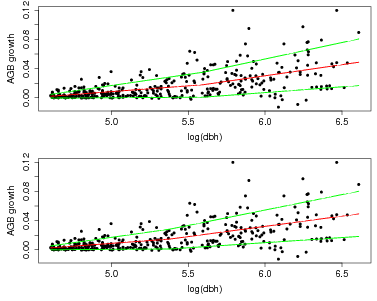There is a function graph.growthmodel.spp that handles the graphing in a single step. It graphs the points for individual trees, and adds the fitted model in blue (you can change the color). A green line shows the mean growth in 10 equally-spaced divisions (from graphdiv=10). There is also an option to overlay alternative model fits as a way of visualizing statistical confidence in the model, illustrated in the second example below (conf=50): 50 different gray lines are drawn using randomly selected parameters from the Gibbs sampler used to fit the model (all the parameters are returned in fit\$fullparam). An advantage of the Gibbs sampler is this way of showing confidence: every set of parameters returned (after the burnin) can be considered equally good fits to the model.

Note that confidence in the model is different than the standard deviation (yellow lines in the previous figure). The latter gives variation among individuals, but the gray lines provide an estimate of confidence in the position of the blue line.

At small dbh (remember, though, only dbh100 mm are included), the SD is small, meaning little variation among individuals, and the confidence bands are narrow. Both SD and the confidence bands spread at larger size.

> meangrowth=graph.growthmodel.spp(fit,regclr='green',modelclr='blue',graphdiv=10,conf=0)
> meangrowth=graph.growthmodel.spp(fit,regclr='green',modelclr='blue',graphdiv=10,conf=50)

Figure 4.2: Growth rate of Tetragastris panamensis, with fit of two-bin model (blue) and estimated growth in 10 equally-spaced log(dbh) bins.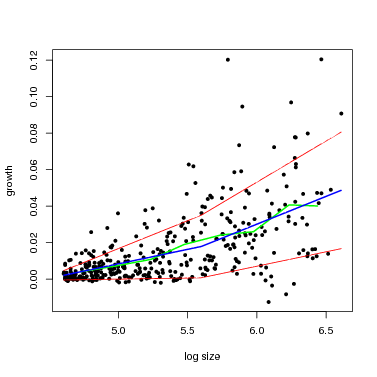Figure 4.3: Growth rate Tetragastris panamensis, with fit of two-bin model (blue) and estimated growth in 10 equally-spaced log(dbh) bins, plus estimated variation in the model given as gray lines.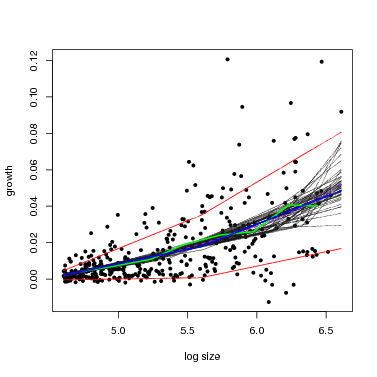4.3. Interpreting the parameters.

The parameters provided by fit\$best describe the model. The first parameter is the break point between the two bins, and the best estimate is 6.04 for in the example here for Tetragastris. The next two parameters are the two slopes, first before the break point, then after the division. In this case, the best fit for them is 0.017 and 0.039, so the slope gets steeper, and both are significantly > 0 (since the confidence intervals do not reach 0). But the two slopes are not significantly different from each other, since their confidence limits overlap. The last parameter is the intercept parameter, which is the estimated growth in the middle of the middle bin.

4.4. Comparing results for different number of bins.

The interesting result is whether using three or four bins improves the model. The function run.growthfit.bin automates the process of running the model 4 times, with 1 through 4 bins. One bin is just a standard linear model; I have not yet tried 5 or more bins.

The following example runs the Gibbs sampler for 1500 repetitions (noreps=1500). In final fits, I recommend using noreps=5000. But for illustration here, the 1500 steps provide adequate parameter values. The variable fitlist creates a list with just one entry, named for the species’ code tet2pa. This is how the data are passed to the graphing function.

> if(!exists('fit1.4'))
+  fit1.4=run.growthfit.bin(growthdata=onespdata,size="agb",startpar=c(.03,.005),
+                           startsdpar=c(.04,0),sdmodel=linear.model.ctr,binoption=1:4,
+                           noreps=1500,noburn=500,noshow=500)
> fitlist=list(tet2pa=fit1.4)

The funtion overlay.growthbinmodel makes a graph of all 4 models. It expects the fit to be a list, which is why I created fitlist. The first option below shows the graph on the screen; the second exports the graph as a pdf (export=pdf ).

> overlay.growthbinmodel(fit=fitlist,bins=1:4,regclr="green",

> overlay.growthbinmodel(fit=fitlist,bins=1:4,regclr="green",
+                        export=pdf,outfile="linearbin.overlay.pdf",h=8,w=10)

Figure 4.4: Growth rate of Tetragastris panamensis with model fits for 1-4 bins. The green line is the average growth in 10 categories. The fit with one bin is blue (that’s just a straight line), for two bins red, three gray, and four black.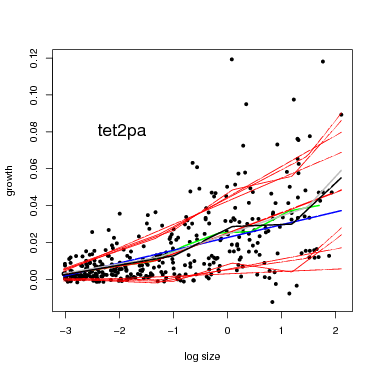4.5. Fitting the model for all species.

The next step is to run the fit for all species in a plot. Here I limit the selction to species with at least 40 individuals total and 15 individuals 300 mm dbh. You may wish to change the limits, but the fitting procedure requires 5 individuals per category, so it will fail with species having < 20 individuals above 100 mm dbh.

This will run the Gibbs sampler 5000 steps per species, and will take 10-20 hours to complete. Note that the example here uses agb as the predictor by submitting size=’agb’.

> attach_if_needed('growth/linearbin.fit.bci.rdata')

> allspecies=sort(unique(agb.growth\$sp))
> linearbin.fit.allspp=
+    run.growthbin.manyspp(growthdata=agb.growth,size='agb',spp=allspecies,
+                          minabund300=15,minTotal=40,dbhunit='mm',
+                          startpar=c(.03,.005),startsdpar=c(.04,0))
> save(linearbin.fit.allspp,file='growth/linearbin.fit.allspp.rdata')

The output, saved in the object linearbin.fit.allspp, is a list, one element per species. Each element has four subelements, one per the models with 1-4 bins. Each of those subelements is a single model fit, with all the elements described above.

The object linearbin.fit.allspp can be passed directly to the function overlay.growthbinmodel to graph results for all the species. In the first example, export=NULL and the graphs are printed to the screen. In the second example, export=pdf and a pdf with all the graphs is created. You can change the file name to whatever you please using outfile.

> overlay.growthbinmodel(fit=linearbin.fit.allspp,bins=1:4,regclr="green",
> overlay.growthbinmodel(fit=linearbin.fit.allspp,bins=1:4,regclr="green",
+                        export=pdf,outfile="linearbin.overlay.pdf",h=8,w=10)

4.6. Statistical comparison of the models.

Finally comes a statistical test for assessing the 4 different models (1-4 bins) for each species. The function compare.growthbinmodel calculates the best model using the AIC, or Akaike Information Criteria, and makes a graph of this model.

It also produces a list of 3 different tables:

1. The first gives the slope of the growth vs. AGB regression in each bin of the best model. There are up to four slopes per species.
2. The second gives the lower 95% confidence limits (or credible intervals in Bayesian terminology) for those same slopes.
3. The third has upper credible intervals.

The results from 37 species at BCI are shown below. The columns with NA indicate those slopes were not needed in the best fit, that is, if there is only one slope, then the best model used only one bin, etc. Any negative slope means growth rate declined with size in that size bin. If the upper credible interval is also negative, then the slope is significantly < 0. At BCI, only quaras, Quararibea asterolepis, had a significant decrease in growth rate in the largest size category. Brosimum alicastrum (brosal) and Prioria copaifera (pri2co). had declines, but not significantly so. Poulsenia armata (poular) had a peculiar fit, with a decline in growth in the second to last category, but an increase in the last. Poulsenia was the only species for which all 4 bins were needed, and only 3 other species required 3 bins. More than half the species (20 or 37) were best modeled using a straight line.

Estimated slopes in AGB bins for BCI species, based on the model with the highest AIC.

> model.compare=compare.growthbinmodel(fit=linearbin.fit.allspp,export=NULL,makegraph=FALSE)
> model.compare\$slopes
[,1]         [,2]        [,3]      [,4]
alchco  0.0032465338           NA          NA        NA
alsebl  0.0021806654  0.007128261  0.10890872        NA
apeime  0.0046786758  0.035849494          NA        NA
aspicr  0.0283336937           NA          NA        NA
beilpe  0.0033653161  0.013751175          NA        NA
brosal  0.0024068701  0.047129124 -0.17796505        NA
casear -0.0253325121  0.008690913          NA        NA
cecrin  0.0050180792           NA          NA        NA
cordal  0.0117999289           NA          NA        NA
cordbi  0.0039835569           NA          NA        NA
dendar  0.0025569194  0.116643536          NA        NA
drypst  0.0036403207  0.018269066          NA        NA
guapst  0.0103665438           NA          NA        NA
guargu  0.0019973357           NA          NA        NA
guatdu -0.0010994482  0.027943355          NA        NA
gustsu  0.0015635930           NA          NA        NA
heisco  0.0011742999           NA          NA        NA
hirttr  0.0017723195           NA          NA        NA
huracr  0.0161435081  1.183355785          NA        NA
jac1co  0.0145098911           NA          NA        NA
loncla  0.0073481019           NA          NA        NA
luehse  0.0079207901           NA          NA        NA
ocotwh  0.0147476415           NA          NA        NA
poular  0.0008762754  0.049263099 -0.08280719 0.1826693
poutre  0.0150712909           NA          NA        NA
pri2co  0.0028850771  0.033175754 -0.02125288        NA
protte  0.0017907969           NA          NA        NA
quaras  0.0067436907 -0.033460006          NA        NA
simaam  0.0059474071  0.027965169          NA        NA
sponra  0.0047933671  0.040976050          NA        NA
tab1ro  0.0069016656  0.166647884          NA        NA
tab2ar  0.0077546177           NA          NA        NA
tet2pa  0.0069909722           NA          NA        NA
tri2tu  0.0039848927  0.012648412          NA        NA
virose  0.0023402584           NA          NA        NA
virosu -0.0060751517  0.017998711          NA        NA
zantbe  0.0241310891           NA          NA        NA

Upper confidence limits for slopes in AGB bins. Any of these < 0 indicate a slope significantly less than zero. There are also lower confidence limits (not shown), and any of those > 0 indicate a significantly positive slope.

> model.compare\$upper
[,1]         [,2]         [,3]      [,4]
alchco  0.005792440           NA           NA        NA
alsebl  0.003896948  0.008517255  0.162687781        NA
apeime  0.005269493  0.116905396           NA        NA
aspicr  0.035465859           NA           NA        NA
beilpe  0.004758150  0.016956525           NA        NA
brosal  0.005181650  0.066313528  0.032898032        NA
casear -0.000402689  0.012374577           NA        NA
cecrin  0.006276610           NA           NA        NA
cordal  0.016520575           NA           NA        NA
cordbi  0.005075125           NA           NA        NA
dendar  0.004150830  0.168619765           NA        NA
drypst  0.004727390  0.027774678           NA        NA
guapst  0.013970905           NA           NA        NA
guargu  0.002744795           NA           NA        NA
guatdu  0.002862032  0.068154978           NA        NA
gustsu  0.001816538           NA           NA        NA
heisco  0.001714928           NA           NA        NA
hirttr  0.002388317           NA           NA        NA
huracr  0.022292374  1.485571198           NA        NA
jac1co  0.016943170           NA           NA        NA
loncla  0.009518829           NA           NA        NA
luehse  0.014597148           NA           NA        NA
ocotwh  0.020653555           NA           NA        NA
poular  0.001844633  0.065679098 -0.008801082 0.2599029
poutre  0.017404537           NA           NA        NA
pri2co  0.005328017  0.040479278  0.010913174        NA
protte  0.002503612           NA           NA        NA
quaras  0.007552048 -0.006168187           NA        NA
simaam  0.008965739  0.039581944           NA        NA
sponra  0.010183281  0.085681413           NA        NA
tab1ro  0.012633114  0.461891000           NA        NA
tab2ar  0.009206953           NA           NA        NA
tet2pa  0.007870435           NA           NA        NA
tri2tu  0.004513265  0.017689708           NA        NA
virose  0.002991947           NA           NA        NA
virosu  0.012829836  0.038371756           NA        NA
zantbe  0.032282730           NA           NA        NA

Graphs of the best model based on AIC for all species.

> attach_if_needed('growth/linearbin.fit.bci.rdata')
 4
> par(mfcol=c(6,3),mai=c(.5,.65,0.25,0.25),cex.lab=1.5,cex.axis=1.5)
> temp=compare.growthbinmodel(fit=linearbin.fit.allspp[1:18],export=NULL,makegraph=TRUE,
+  newgraph=FALSE,conf=0)
> attach_if_needed('growth/linearbin.fit.bci.rdata')
 4
> par(mfcol=c(7,3),mai=c(.5,.65,0.25,0.25),cex.lab=1.5,cex.axis=1.5)
> temp=compare.growthbinmodel(fit=linearbin.fit.allspp[19:37],export=NULL,makegraph=TRUE,
+                        newgraph=FALSE,conf=0)

Figure 4.5: Growth rates of all 37 species fitted with simple SD model and strict trimming. The gray lines indicate confidence by giving 20 alternate fits from the Gibbs sampler.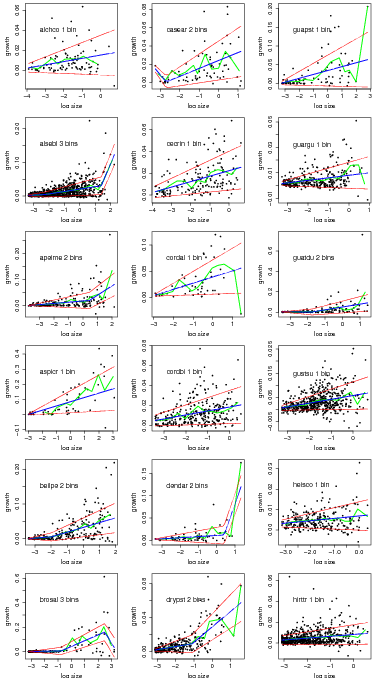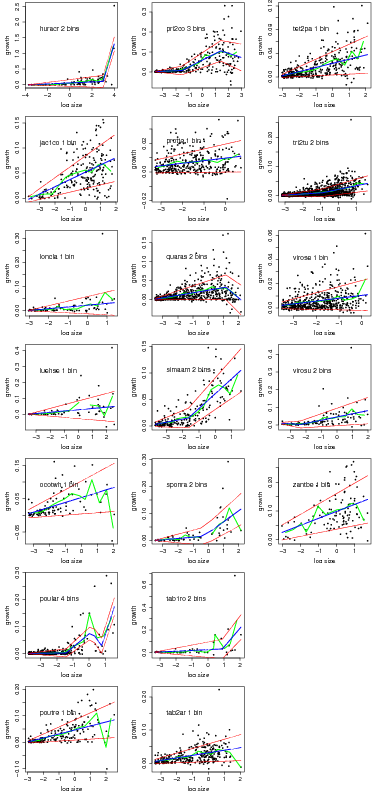Now use a run with an updated trimming of extreme growth, permitting more negative growth (see trimGrowth), and the alternative model of SD where the slope can turn upward at large size.

> if(!exists('trimmed3'))
+  trimmed3=extract.growthdata(census1=cns1,census2=cns2,growcol='incgr',growthfunc=growth.biomass.indiv,
+                              logit='x',rounddown = FALSE,pomcut=.05,mindbh = 100,
+                              dbhunit = 'mm',err.limit = 12,maxgrow = 40)
> if(!exists('linearbin.fit.trim3'))
+ linearbin.fit.trim3=
+  run.growthbin.manyspp(growthdata=trimmed3,size='agb',spp=allspecies,minabund300=15,
+                        minTotal=40,dbhunit='mm',startpar=c(.03,.005),startsdpar=c(.04,0,.001),
> model.compare=compare.growthbinmodel(fit=linearbin.fit.trim3,export=NULL,makegraph=FALSE)
> model.compare\$slopes
[,1]         [,2]      [,3] [,4]
alchco  2.313291e-03           NA        NA   NA
alsebl  8.801125e-04  0.006729967 0.1165375   NA
apeime  5.377643e-03           NA        NA   NA
aspicr  7.659387e-03  0.056497966        NA   NA
beilpe  3.434838e-03  0.014251442        NA   NA
brosal -9.937160e-06  0.045653429        NA   NA
casear  6.236175e-03           NA        NA   NA
cecrin  4.344572e-03           NA        NA   NA
chr2ar  4.342516e-04           NA        NA   NA
cordal  1.119337e-02           NA        NA   NA
cordbi  3.850902e-03           NA        NA   NA
dendar  3.061239e-03  0.103986771        NA   NA
drypst  4.046738e-03           NA        NA   NA
guapst  8.774790e-03           NA        NA   NA
guargu  1.933300e-03  0.016178454        NA   NA
guatdu -3.233411e-04  0.012647192        NA   NA
gustsu  1.484349e-03           NA        NA   NA
heisco  1.037609e-03           NA        NA   NA
hirttr  1.787888e-03           NA        NA   NA
huracr  7.576259e-04  0.076699153 0.1477084   NA
ingama  1.218236e-02           NA        NA   NA
jac1co  1.558190e-02           NA        NA   NA
loncla  4.174202e-03           NA        NA   NA
luehse  6.472729e-03           NA        NA   NA
ocotwh  1.638501e-02 -1.507224977        NA   NA
poular  1.232570e-03  0.036611405        NA   NA
poutre  5.097094e-03  0.017228969        NA   NA
pri2co  3.300787e-03  0.025710413        NA   NA
protte  1.633665e-03           NA        NA   NA
quaras  6.211712e-03           NA        NA   NA
simaam  6.996646e-03  0.029710478        NA   NA
sponra  3.689212e-03  0.038736659        NA   NA
tab1ro  7.552564e-04           NA        NA   NA
tab2ar  7.830165e-03           NA        NA   NA
tachve  4.926326e-03  0.128908115        NA   NA
tet2pa  6.873703e-03           NA        NA   NA
tri2tu  2.661230e-03  0.008013637        NA   NA
virose  2.286633e-03           NA        NA   NA
virosu  7.480564e-04  0.019794913        NA   NA
zantbe  1.879699e-02           NA        NA   NA
> model.compare\$upper
[,1]         [,2]      [,3] [,4]
alchco 0.0047805797           NA        NA   NA
alsebl 0.0026159073  0.007851163 0.1842141   NA
apeime 0.0071833650           NA        NA   NA
aspicr 0.0191826934  0.080957890        NA   NA
beilpe 0.0057810590  0.017617172        NA   NA
brosal 0.0006205874  0.073233290        NA   NA
casear 0.0086761343           NA        NA   NA
cecrin 0.0063719731           NA        NA   NA
chr2ar 0.0024420043           NA        NA   NA
cordal 0.0153462792           NA        NA   NA
cordbi 0.0052195064           NA        NA   NA
dendar 0.0051950156  0.176018295        NA   NA
drypst 0.0052035559           NA        NA   NA
guapst 0.0130986123           NA        NA   NA
guargu 0.0028043138  0.069607345        NA   NA
guatdu 0.0023154959  0.018383915        NA   NA
gustsu 0.0018811924           NA        NA   NA
heisco 0.0016746418           NA        NA   NA
hirttr 0.0023245174           NA        NA   NA
huracr 0.0028343234  0.107355627 0.6054979   NA
ingama 0.0223377262           NA        NA   NA
jac1co 0.0174474237           NA        NA   NA
loncla 0.0073316976           NA        NA   NA
luehse 0.0107542164           NA        NA   NA
ocotwh 0.0222512241 -0.322498697        NA   NA
poular 0.0018242545  0.051693888        NA   NA
poutre 0.0140629751  0.025875535        NA   NA
pri2co 0.0051542918  0.031276790        NA   NA
protte 0.0024564011           NA        NA   NA
quaras 0.0070888172           NA        NA   NA
simaam 0.0096095343  0.040682545        NA   NA
sponra 0.0076408366  0.091596492        NA   NA
tab1ro 0.0038115804           NA        NA   NA
tab2ar 0.0092720217           NA        NA   NA
tachve 0.0116175257  0.250464577        NA   NA
tet2pa 0.0082352449           NA        NA   NA
tri2tu 0.0039823663  0.009757620        NA   NA
virose 0.0029423020           NA        NA   NA
virosu 0.0063641490  0.063036954        NA   NA
zantbe 0.0256678498           NA        NA   NA

Graphs of the best model based on AIC for all species.

> attach_if_needed('growth/linearbin.fittrim3.bci.rdata')
 2
> par(mfcol=c(7,3),mai=c(.5,.65,0.25,0.25),cex.lab=1.5,cex.axis=1.5)
> temp=compare.growthbinmodel(fit=linearbin.fit.trim3[1:21],export=NULL,makegraph=TRUE,
+                             newgraph=FALSE,conf=0)
> par(mfcol=c(6,3),mai=c(.5,.65,0.25,0.25),cex.lab=1.5,cex.axis=1.5)
> temp=compare.growthbinmodel(fit=linearbin.fit.trim3[22:40],export=NULL,makegraph=TRUE,
+                             newgraph=FALSE,conf=0)

Figure 4.6: Growth rates of all 40 species fitted with simple SD model and strict trimming. The gray lines indicate confidence by giving 20 alternate fits from the Gibbs sampler.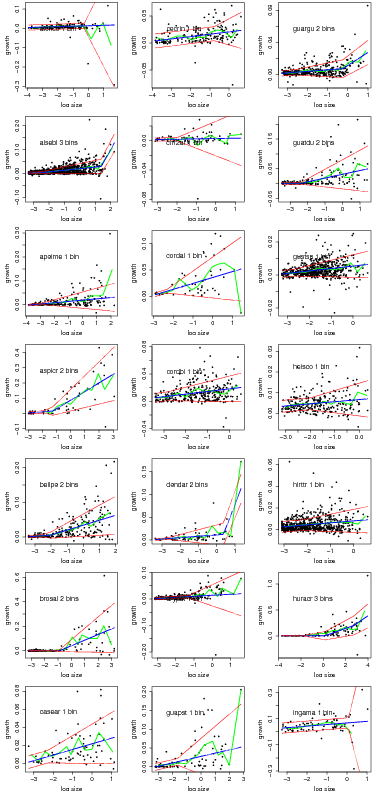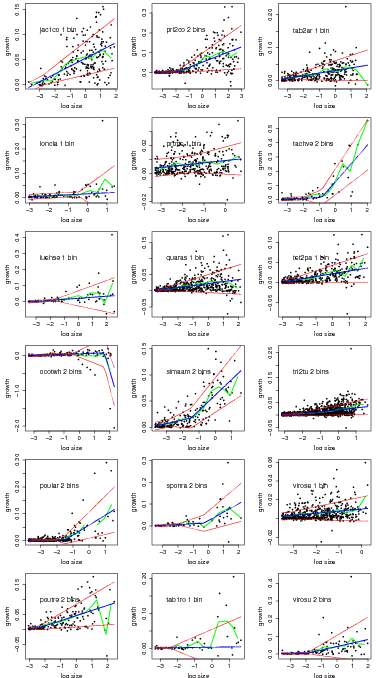You can also export all the graphs at once into a single pdf to a file named by outfile.

> temp=compare.growthbinmodel(fit=linearbin.fit.allspp,export=pdf,makegraph=TRUE,
+                            newgraph=FALSE,outfile="linearbin.orig.pdf")
> temp=compare.growthbinmodel(fit=linearbin.fit.trim3,export=pdf,makegraph=TRUE,
+                            newgraph=FALSE,outfile="linearbin.trim3.pdf")

### 5. File creation

Anyone intereted in seeing how Sweave works in R to create this document can contact me.

> Sweave(file="growth/growthFitBin.Rnw",keep.source=TRUE);
> system("pdflatex growthFitBin");
> system("htlatex growthFitBin");
> system("rm ~/data/growth/growthfitAnalysis/*")
> system("mv ~/data/growthFitBin* ~/data/growth/growthfitAnalysis/");
> system("mv ~/data/growth/growthfitAnalysis/growthFitBin.html ~/data/growth/growthfitAnalysis/index.html");
> system("rsync -ave ssh ~/data/growth/growthfitAnalysis rcondit@ctfs.arnarb.harvard.edu:/var/www/html/Public/CTFSRPackage/tutorials/ --whole-file");
> system("cp -r ~/data/growth/growthfitAnalysis /var/www/Public/CTFS_RPackage/files/tutorials/")